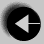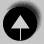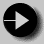## Assignment Statement

The easiest example of an expression is in the assignment statement. An expression is evaluated, and the result is saved in a variable. A simple example might look like

```
y = (m * x) + c```

This assignment will save the value of the expression in variable y.Go to ExpressionsGo to IndexGo to Arithmetic Operators